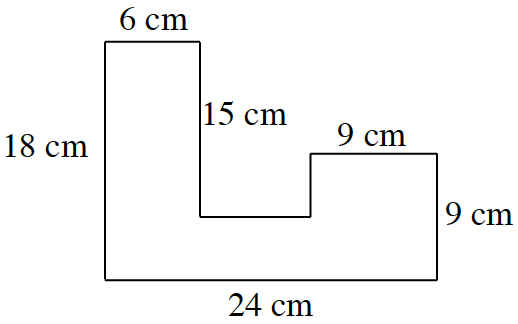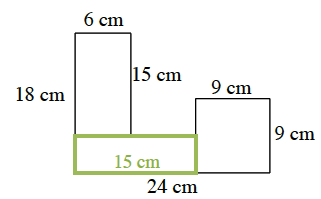### Home > ACC6 > Chapter 8 Unit 8 > Lesson CC1: 8.1.4 > Problem8-54

8-54.

Find the area of the shape below. All angles are right angles.To find the area of the entire shape, separate it into rectangles.

One way to divide the shape up is to make a $6$ cm by $15$ cm rectangle, a $9$ cm by $9$ cm square, and a rectangle with unknown sides. To find its length, you must subtract the $9$ cm side from the $24$ cm parallel to it. To find its width, you must subtract the vertical $15$cm side from $18$ cm side.$24\text{ cm}-9\text{ cm}=15\text{ cm}$
$18\text{ cm}-15\text{ cm}=3\text{ cm}$

Find the areas of the rectangles and add them together.

$(6 \text{ cm} × 15 \text { cm}) + (9 \text{ cm} × 9 \text{ cm}) + (15 \text{ cm} × 3 \text{ cm}) = ?$

The area of the shape is $216$ square centimeters.